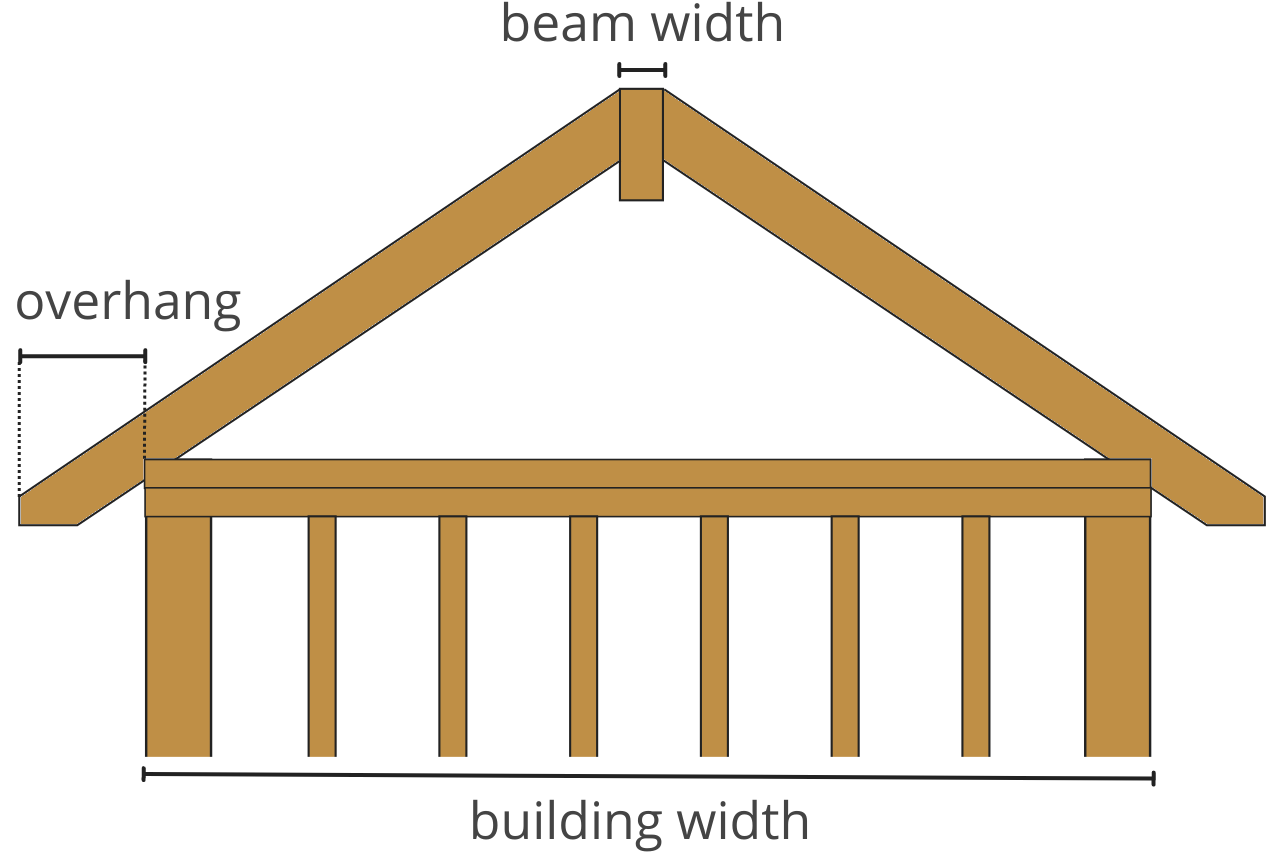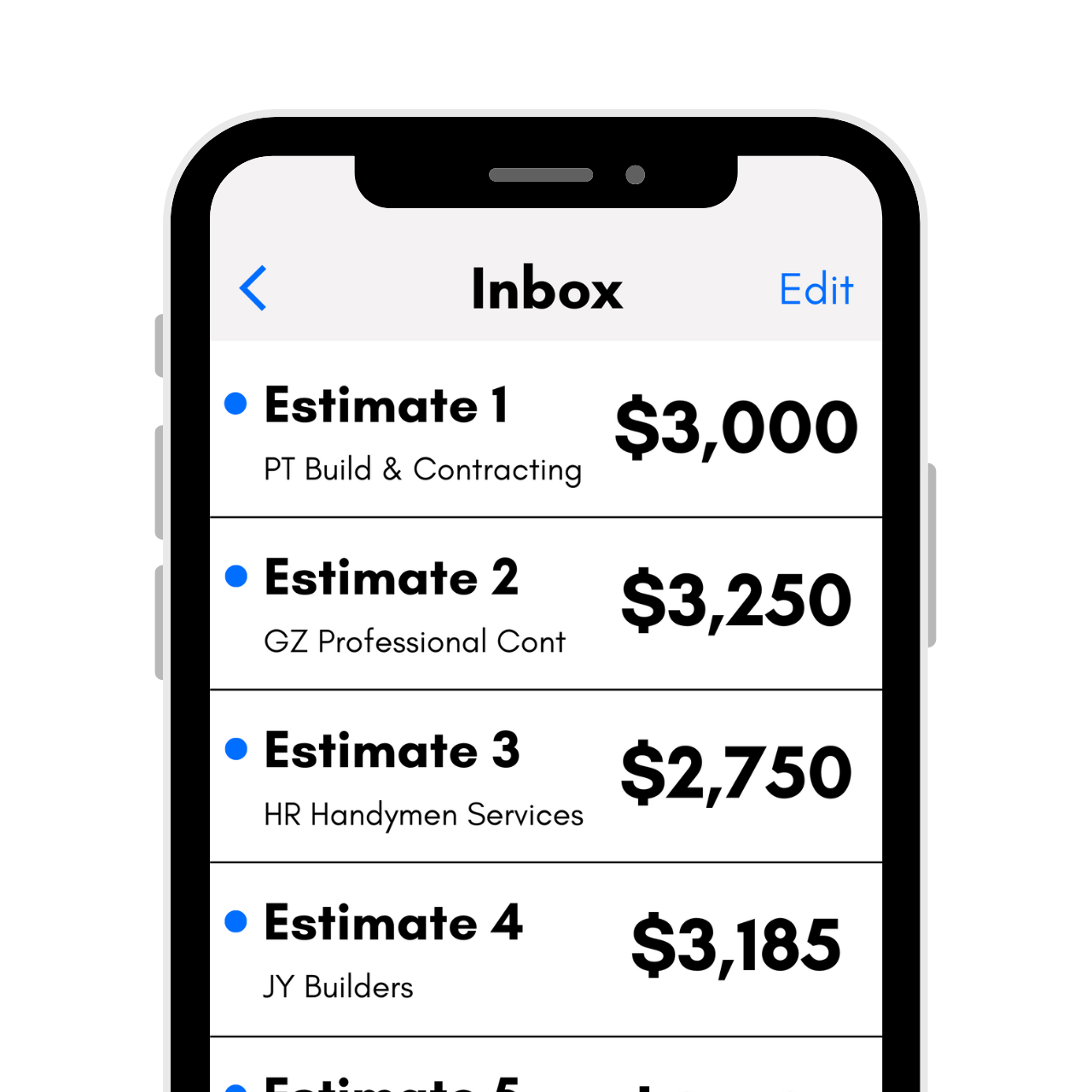# Rafter Length Calculator

Calculate the length of a rafter given the width of the building, overhang, and width of the beam, if any.find roof pitch

## Rafter Length:

 Feet and Inches: Feet: Inches:

### Roof Details

 Roof Angle: Slope Factor: Hip & Valley Factor:
Learn how we calculated this below

## How to Calculate the Length of a Rafter

A rafter is a structural member that provides support for the roof sheathing and transfers the weight of the roof to the exterior walls of a building. Calculating the length of the rafters is an essential part of framing a roof.

There are many different types of rafters, and the type you use will be influenced by the type of roof. For example, dormer roofs will require dormer rafters in addition to common rafters, while hip rafters will be required for hipped roofs.

The length of a rafter is the distance between the point where the rafter meets the beam (or opposing rafter for a truss) and the end of the overhang. Note that this is not necessarily the same as the length of the rafter board, which might need to be cut longer to account for rafter tails or an edge profile.

Each type of rafter may need to be measured differently. You can calculate the length of a common rafter in a few easy steps.

Get free estimates from carpentry pros in your area to compare prices### Step One: Calculate the Roof Pitch

The roof pitch is the angle of the roof and can be measured in several ways, but is most commonly expressed in rise over a standard 12-inch run. But, in order to calculate the rafter length, we need to calculate the pitch in degrees.

The angle in degrees is equal to the inverse tangent of the pitch of the roof.

angle = arctan(pitch / 12)

You can also find the angle for your roof pitch in the table below.

Table showing the angle in degrees for various roof pitches.
Roof Pitch Angle in Degrees
0/12
1/12 4.76°
2/12 9.46°
3/12 14.04°
4/12 18.43°
5/12 22.62°
6/12 26.57°
7/12 30.26°
8/12 33.69°
9/12 36.87°
10/12 39.81°
11/12 42.51°
12/12 45°

Pitch can be used to help calculate the rise. This is the total height of the roof. If you have this figure, you can use it plus the run, to get the rafter length.

The rise is also the term frequently used for the vertical beam that the rafters will butt against. You can use the following formula to get the rise:

rise = run × pitch

The run is half the measure of the roof span. You can also use our rise over run calculator to find this.

### Step Two: Measure the Roof Span

The roof’s span is the total length of the roof. You’ll also need to account for the overhang beyond the building on either side.

Measure the span by measuring the width of the building using a tape measure, then add the overhang on each side to the measurement.

### Step Three: Calculate the Rafter Run

The rafter’s run is the horizontal distance between the end of the rafter and where it meets the beam, which is known as the rise. To calculate the run, divide the total width of the building in half. Then, account for the beam or ridge board by subtracting half of its width from the run.

run = (total width ÷ 2) – (beam width ÷ 2)

### Step Four: Calculate the Rafter Length

Now that you know the run and the angle in degrees, you can use trigonometry to calculate the rafter length. Since the cosine of an angle in a right triangle is equal to the length of the adjacent side divided by the hypotenuse, we can derive the following formula to calculate the rafter length:

rafter length = run ÷ cos(angle)

The rafter length is equal to the run divided by the cosine of the roof’s angle.

Another method is to use the rise of the roof using this formula:

rise² + run² = rafter length²

Meaning that your rafter length will equal:

rafter length = √(rise² + run²)

Remember that things like allowance, lumber size, and roof type can play into the overall length of each rafter.

You might also be interested in our roofing calculator to find how many squares of shingles you’ll need to finish it off.